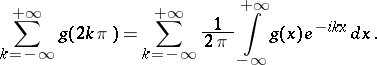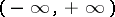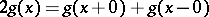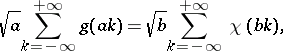# Poisson summation formulaThe Poisson summation formula holds if, for example, the functionis absolutely integrable on the interval, has bounded variation and. The Poisson summation formula can also be written in the formwhereandare any two positive numbers satisfying the condition, andis the Fourier transform of the function: